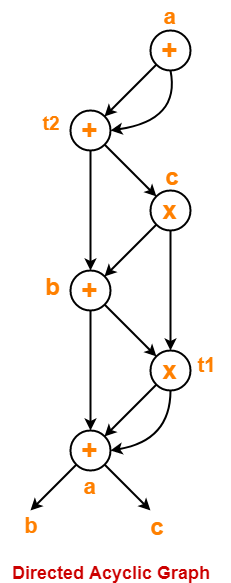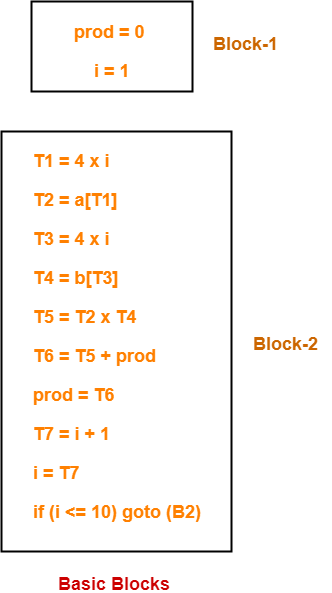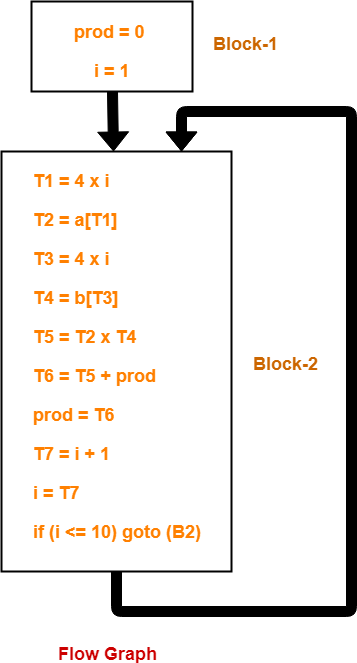## Three Address Code | DAGs | Basic Blocks & Flow Graphs-

Before you go through this article, make sure that you have gone through the previous articles on-

In this article, we will solve Miscellaneous Problems based on these Concepts.

## Problem-01:

Generate three address code for the following code-

c = 0

do

{

if (a < b) then

x++;

else

x–;

c++;

} while (c < 5)

## Solution-

Three address code for the given code is-

1. c = 0
2. if (a < b) goto (4)
3. goto (7)
4. T1 = x + 1
5. x = T1
6. goto (9)
7. T2 = x – 1
8. x = T2
9. T3 = c + 1
10. c = T3
11. if (c < 5) goto (2)

## Problem-02:

Generate three address code for the following code-

while (A < C and B > D) do

if A = 1 then C = C + 1

else

while A <= D

do A = A + B

## Solution-

Three address code for the given code is-

1. if (A < C) goto (3)
2. goto (15)
3. if (B > D) goto (5)
4. goto (15)
5. if (A = 1) goto (7)
6. goto (10)
7. T1 = c + 1
8. c = T1
9. goto (1)
10. if (A <= D) goto (12)
11. goto (1)
12. T2 = A + B
13. A = T2
14. goto (10)

## Problem-03:

Generate three address code for the following code-

switch (ch)

{

case 1 : c = a + b;

break;

case 2 : c = a – b;

break;

}

## Solution-

Three address code for the given code is-

if ch = 1 goto L1

if ch = 2 goto L2

L1:

T1 = a + b

c = T1

goto Last

L2:

T1 = a – b

c = T2

goto Last

Last:

## Problem-04:

Construct a DAG for the following three address code-

1. a = b + c
2. t1 = a x a
3. b = t1 + a
4. c = t1 x b
5. t2 = c + b
6. a = t2 + t2

## Solution-

Directed acyclic graph for the given three address code is-## Problem-05:

Consider the following code-

prod = 0 ;

i = 1 ;

do

{

prod = prod + a[ i ] x b[ i ] ;

i = i + 1 ;

} while (i <= 10) ;

1. Compute the three address code.
2. Compute the basic blocks and draw the flow graph.

## Solution-

### Part-01:

Three address code for the given code is-

prod = 0

i = 1

T1 = 4 x i

T2 = a[T1]

T3 = 4 x i

T4 = b[T3]

T5 = T2 x T4

T6 = T5 + prod

prod = T6

T7 = i + 1

i = T7

if (i <= 10) goto (3)

### Step-01:

We identify the leader statements as-

• prod = 0 is a leader because first statement is a leader.
• T1 = 4 x i is a leader because target of conditional or unconditional goto is a leader.

### Step-02:

The above generated three address code can be partitioned into 2 basic blocks as-### Step-03:

The flow graph is-To gain better understanding about these Miscellaneous Problems,

Watch this Video Lecture